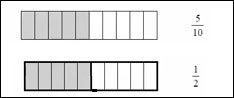# Introduction to Simplifying a Fraction

A fraction is said to be in simplest form if the highest common factor of its numerator and denominator is 1. A given fraction may be or may not be in the simplest form.

To simplify a fraction or to reduce it to its simplest form, we find the highest common factor (hcf) of both its numerator and denominator. The fraction is reduced to its simplest form by dividing its numerator and denominator by hcf found.

Write 5/10 in the simplest form.

### Solution

Step 1:

We find the greatest number that divides both 5 and 10 exactly is 5. We use it to simplify the fraction as shown below.

5/10 = (5 ÷ 5) / (10 ÷ 5) = 1/2

Step 2:

The fraction 1/2 is in simplest from. The answer is 1/2.

Step 3:

Below 5 out of 10 bars are shaded.Step 4:

We can make the bars into two equal parts. We see that 1 of these 2 parts is shaded. Therefore 5/10 = 1/2

Write 4/16 in simplest form.

### Solution

Step 1:

We find the greatest number that divides both 4 and 16 exactly is 4. We use it to simplify the fraction as shown below.

4/16 = 4/16 ÷ 4/4 = (4 ÷ 4) / (16 ÷ 4) = 1/4

Step 2:

The fraction 1/4 is in simplest from. The answer is 1/4.

Write 9/12 in simplest form.

### Solution

Step 1:

We find the greatest number that divides both 9 and 12 exactly is 3. We use it to simplify the fraction as shown below.

9/12 = 9/12 ÷ 3/3 = (9 ÷ 3) / (12 ÷ 3) = 3/4

Step 2:

The fraction 3/4 is in simplest from. The answer is 3/4.